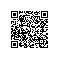# 把99%的程序员烤得外焦里嫩的JavaScript面试题

var a = 10;
{

a = 99;
function a() {
}

a = 30;

}
console.log(a);

var a = 10;
{

function hello() {
a = 99;
function a() {
}

a = 30;
}
hello();

}
console.log(a);

1. 执行级代码块和非执行级代码块

{

  var a = 1;
var b = 2;
console.log(a + b);

}

{

var a = 1;
var b = 2;
console.log(a + b);

}

1. 执行级代码块中的变量和函数自动提升作用域
2. 如果有局部符号，执行级代码块会优先进行作用域提升，而非执行级代码块，会优先考虑局部符号

（1）执行级代码块中的变量和函数自动提升作用域

{

var a = 1;
var b = 2;
function sub() {
return a - b
}

}

console.log(a + b); // 输出3
console.log(sub()); // 输出-1

var a = 14;
function b() {

}
{

var a = 1;

var b = 2;
function sub() {
return a - b
}

}

console.log(a + b); // 输出3
console.log(sub()) // 输出-1

let a = 14;
class b{}
{

var a = 1;

var b = 2;
function sub() {
return a - b
}

}

console.log(a + b);
console.log(sub())

function myfun()
{

var a = 1;
var b = 2;

}
console.log(a + b);

（2）如果有局部符号，执行级代码块会优先进行作用域提升，而非执行级代码块，会优先考虑局部符号，看下面的解释。

var a = 100
{

a = 10;
function a() {

}
a = 20;


}
console.log(a)； // 输出10

var a = 100
{

function hello() {
a = 10;
function a() {

}

a = 20;
}
hello();


}
console.log(a)； // 输出100

1. 多遍扫描

function hello() {
}
hello()

hello()
// hello函数是在使用之后定义的
function hello() {
}

hello()
function hello() {
}

hello()
function hello() {

console.log('hello')

}

var h = new hello(); // 抛出异常
class hello {

}

class hello {
}
var h = new hello(); // 正常创建类的实例

var p1 = 10
{

p1 = 40;
class p1{}
p1 = 50;

}

1. 下面哪段代码会抛出异常

var a = 99;
function a() {
}
console.log(a)

{

var a = 99;
function a() {
}
console.log(a)

}

{

a = 99;
function a() {
}
console.log(a)

}

function hello()
{

var a = 99;
function a() {
}
console.log(a)

}
hello();

var a = 99;
function a() {
}
console.log(a)

function hello()
{

var a = 99;
function a() {
}
console.log(a)

}
hello();

{

var a = 99;          // 抛出异常
function a() {
}
console.log(a)

}

{

a = 99;           // 正常执行
function a() {
}
console.log(a)

}

1. 定义变量使用var。如果发现块内有同名函数或类定义，会抛出重定义异常
2. 未使用var定义变量。遇到同名函数，函数将被永久覆盖，如果遇到同名类，会抛出如下异常：

var a = 10; // 不处理
{

a = 99;    // 不处理
function a() {   // 提升作用域到顶层作用域
}

a = 30;        // 不处理

}
console.log(a); // 不处理

// 在第2遍扫描时，其实已经发现在第1遍扫描中存在一个顶层的函数a（作用域被提升的），所以这个变量a其实是覆盖了第1遍扫描时的a函数
// 所以说，不是函数a覆盖了变量a，而是变量a覆盖了函数a。也就是说，当执行到这时，函数a已经被干掉了，以后再也没函数a什么事了
var a = 10;
{

a = 99;    // 提升作用域，将a的值设为99，在这时还没有局部函数a呢！
// 在第2遍扫描时仍然处理，由于第1遍扫描，只扫描函数，所以是没有顶级变量a的，因此，会将函数a提升到顶级作用域
// 而第2遍扫描，由于存在顶级变量a，所以这个函数a会作为局部函数处理，这是执行级代码块的规则
function a() {
}

a = 30;       // 实际上替换的是局部函数a

}
console.log(a); // 第2遍执行这条语句，输出99

var a = 10; // 不处理
{

function hello() {        // 提升到顶级作用域
a = 99;               // 不处理
function a() {        // 添加到hello函数作用域的符号表中
}
a = 30;               // 不处理
}
hello();                 // 不处理 

}
console.log(a); // 不处理

var a = 10; // 定义顶层变量a
{

function hello() {        // 提升到顶级作用域
a = 99;               // 如果是非执行级代码块，会优先考虑局部同名符号，如局部函数a，因此，这里实际上覆盖的是函数a，而不是全局变量10
function a() {        // 在非执行级代码块中，只在第1遍扫描中处理内嵌函数，第2遍扫描不处理，所以这是函数a已经被a=99覆盖了
}
a = 30;               // 覆盖a = 99   在hello函数内部，a的最终值是30
}
hello();                 // 执行

}
console.log(a); // 输出10使用钉钉扫一扫加入圈子
+ 订阅